Data analysis using Pandas

• Difficulty Level : Medium
• Last Updated : 15 Oct, 2020

Pandas is the most popular python library that is used for data analysis. It provides highly optimized performance with back-end source code is purely written in C or Python.

We can analyze data in pandas with:

1. Series
2. DataFrames

Series:

Series is one dimensional(1-D) array defined in pandas that can be used to store any data type.

Code #1: Creating Series

 # Program to create series  # Import Panda Libraryimport pandas as pd    # Create series with Data, and Indexa = pd.Series(Data, index = Index)

Here, Data can be:

1. A Scalar value which can be integerValue, string
2. A Python Dictionary which can be Key, Value pair
3. A Ndarray

Note: Index by default is from 0, 1, 2, …(n-1) where n is length of data.

Code #2: When Data contains scalar values

 # Program to Create series with scalar values   # Numeric dataData =[1, 3, 4, 5, 6, 2, 9]    # Creating series with default index valuess = pd.Series(Data)      # predefined index valuesIndex =['a', 'b', 'c', 'd', 'e', 'f', 'g']   # Creating series with predefined index valuessi = pd.Series(Data, Index)

Output: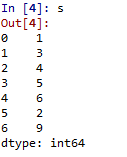Scalar Data with default Index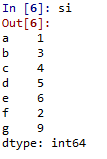Scalar Data with Index

Code #3: When Data contains Dictionary

 # Program to Create Dictionary seriesdictionary ={'a':1, 'b':2, 'c':3, 'd':4, 'e':5}   # Creating series of Dictionary typesd = pd.Series(dictionary)

Output: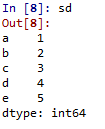Dictionary type data

Code #4:When Data contains Ndarray

 # Program to Create ndarray series  # Defining 2darrayData =[[2, 3, 4], [5, 6, 7]]    # Creating series of 2darraysnd = pd.Series(Data)

Output: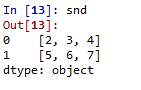Data as Ndarray

DataFrames:

DataFrames is two-dimensional(2-D) data structure defined in pandas which consists of rows and columns.

Code #1: Creation of DataFrame

 # Program to Create DataFrame  # Import Libraryimport pandas as pd     # Create DataFrame with Dataa = pd.DataFrame(Data)

Here, Data can be:

1. One or more dictionaries
2. One or more Series
3. 2D-numpy Ndarray

Code #2: When Data is Dictionaries

 # Program to Create Data Frame with two dictionaries  # Define Dictionary 1dict1 ={'a':1, 'b':2, 'c':3, 'd':4}     # Define Dictionary 2     dict2 ={'a':5, 'b':6, 'c':7, 'd':8, 'e':9}   # Define Data with dict1 and dict2Data = {'first':dict1, 'second':dict2}   # Create DataFrame df = pd.DataFrame(Data)

Output: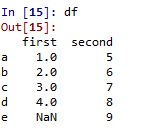DataFrame with two dictionaries

Code #3: When Data is Series

 # Program to create Dataframe of three series import pandas as pd  # Define series 1s1 = pd.Series([1, 3, 4, 5, 6, 2, 9])     # Define series 2       s2 = pd.Series([1.1, 3.5, 4.7, 5.8, 2.9, 9.3])   # Define series 3s3 = pd.Series(['a', 'b', 'c', 'd', 'e'])       # Define DataData ={'first':s1, 'second':s2, 'third':s3}   # Create DataFramedfseries = pd.DataFrame(Data)

Output: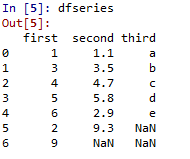DataFrame with three series

Code #4: When Data is 2D-numpy ndarray
Note: One constraint has to be maintained while creating DataFrame of 2D arrays – Dimensions of 2D array must be same.

 # Program to create DataFrame from 2D array  # Import Libraryimport pandas as pd   # Define 2d array 1d1 =[[2, 3, 4], [5, 6, 7]]   # Define 2d array 2d2 =[[2, 4, 8], [1, 3, 9]]   # Define DataData ={'first': d1, 'second': d2}    # Create DataFramedf2d = pd.DataFrame(Data)

Output: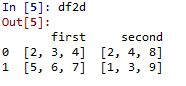DataFrame with 2d ndarray

My Personal Notes arrow_drop_up